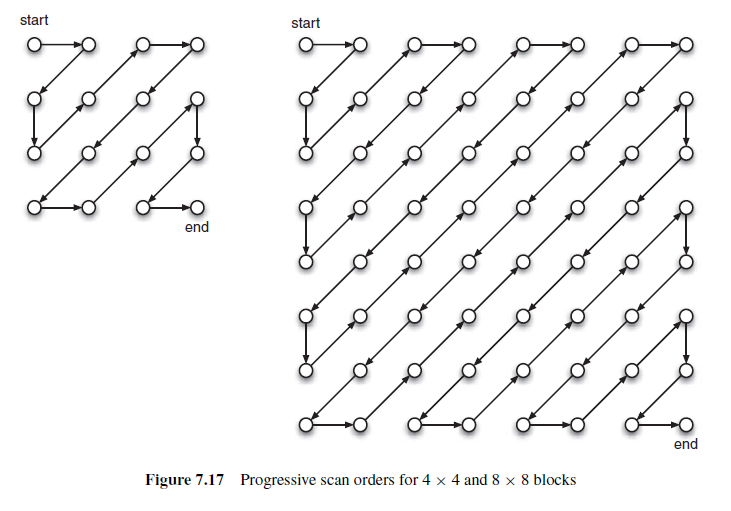# X264源码解析之x264_zigzag_init函数

X264 中关于 zigzag 的函数定义在dct.cx264_zigzag_init中。

Blocks of transform coefficients are scanned, i.e. converted to linear array, prior to entropy coding. The scan order is intended to group together significant coefficients, i.e. non-zero quantized coefficients. In a typical block in a progressive frame, non-zero coefficients tend to be clustered around the top left'DC' coefficient. In this case, a zigzag scan order may be the most efficient, shown in 4x4 and 8x8 blocks. After scanning the block in a zigzag order, the coefficients are placed in a linear array in which most of the non-zero coefficients tend to occur near the start of the array.

However, in an interlaced field or a field of a progressive frame converted from interlaced content, vertical frequencies in each block tend to dominate because the field is vertically sub-sampled from the original scene. This means that non-zero coefficients ten to occur at the top and towards the left side of the block. A block in a field macroblock is therefore scanned in a modified field scan order.

Block scan orders